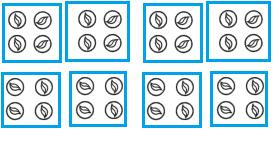Go through the Math in Focus Grade 1 Workbook Answer Key End-of-Year Review to finish your assignments.

Multiple Choice

Fill in the circle next to the correct answer.

Question 1.
Which month has less than 31 days?
(A) January
(B) March
(C) April
(D) October
Option(C)
Explanation:
January, March and October has 31 days,
while April has 30 days.

Question 2.
Nina works from Wednesday to the following Monday. How many days does she work?
(A) 5
(B) 6
(C) 1
(D) 8
Option(B)
Explanation:
1 week has 7 days, from Monday to Sunday
Nina works from Wednesday to the following Monday.
So, she works for 6 days.

Question 3.
_________ is the season that comes after Spring.
(A) Summer
(B) Hot
(C) Winter
(D) Fall
Option(A)
Explanation:
Summer (Grishma Ritu) comes after spring season.
In the northern hemisphere, the summer months are considered June, July and August;
however, in the southern hemisphere, the summer months are December, January and February.

Question 4.
The _________ has a value of 5¢.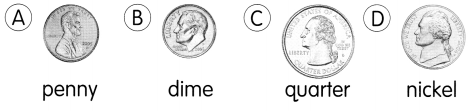Option(D)
Explanation:
1 nickel = 5¢
1 dime = 10¢
1 penny = 1¢
1 quarter = 25¢
So, the nickel has the value of 5¢.

Question 5.
What is the value of the coins?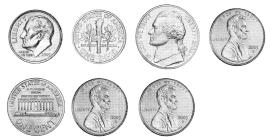(A) 7¢
(B) 19¢
(C) 29¢
(D) 34¢
Option(C)
Explanation:
2 dimes + 1 nickel + 4 pennies
1 nickel = 5¢
1 dime = 10¢
1 penny = 1¢
20¢ + 5¢ + 4¢ = 29¢

Question 6.
This is the money Shari has.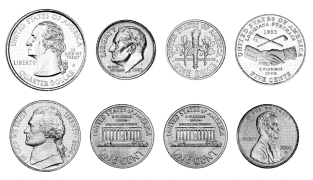She buys a red ribbon for 15¢. How much money does she have left?
(A) 43¢
(B) 53¢
(C) 50¢
(D) 87¢
Option(A)
Explanation:
Shari has total amount of 1 quarter + 2 dimes + 2 nickels + 3 pennies
= 25¢ + 10¢ + 10¢ + 5¢ + 5¢ + 1¢ + 1¢+ 1¢
= 58¢
She buys a red ribbon for 15¢.
Total money left with her after purchasing
58¢ – 15¢ = 43¢

Question 7.
55¢ + 12¢ = ?
(A) 35¢
(B) 53¢
(C) 67¢
(D) 75¢
Option(C)
Explanation:
55¢ + 12¢ = 67¢

Question 8.
The third Friday in July is July 17. What will the fourth Friday be?
(A) July 24
(B) July 22
(C) July 15
(D) August 1
Option(A)
Explanation:
There are 7 days in 1 week.
They repeat after every 7 days.
So, fourth Friday will be on July 24.

Question 9.
65 comes just before ___________.
(A) 55
(B) 64
(C) 66
(D) 75
Option(C)
Explanation:
65 is a successor of 66.
So, 65 comes just before 66.

Question 10.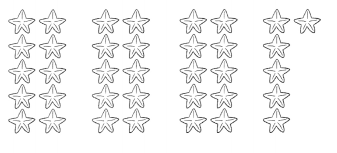3 tens and 6 ones is the same as ___________.
(A) 8
(B) 11
(C) 36
(D) 83
Option(C)
Explanation:
3 tens means 30
6 ones means 6
So, 30 + 6 = 36

Question 11.
Look at the number pattern. Which number comes next?
28, 31, 34, 37, 40 ___________
(A) 31
(B) 41
(C) 43
(D) 50
Option(C)
Explanation:
Rule n + 3
n is the first number of the given series.
28 + 3 = 31
31 + 3 = 34
34 + 3 = 37
37 + 3 = 40
40 + 3 = 43

Question 12.
10 more than 65 is ___________.
(A) 55
(B) 56
(C) 85
(D) 75
Option(D)
Explanation:
Adding 10 to 65 makes 75.
so, 10 more than 65 is 75.

Question 13.
(A) 13
(B) 63
(C) 73
(D) 83
Option(C)
Explanation:
The sum is always greater the addends.
16 + 57 = 73

Question 14.
Find the missing number.
__________ 15, 25, 35, 45
(A) 0
(B) 5
(C) 10
(D) 55
Option(C)
Explanation:
The given series is a multiple of 5
so, the missing number is 10.

Question 15.
Subtract.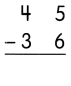(A) 9
(B) 11
(C) 19
(D) 81
Option(A)
Explanation: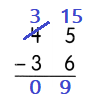Subtract the smaller number from bigger number.
The number 5 in ones place is less than 6,
so borrow 1 from the 4 of tens place.
Regroup ones and tens to find the difference.

Question 16.
Add mentally. 15 + 24 = ____________
(A) 7
(B) 13
(C) 39
(D) 35
Option(C)
Explanation:
10 + 20 = 30.
5 + 4 = 9.
Finally, we add the sum of the tens sum and the sum of the ones:
30 + 9 = 39.
Therefore, 15 + 24 = 39.

Question 17.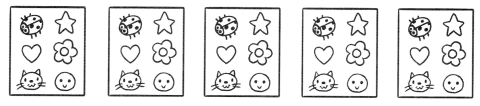There are __________ stickers in all.
(A) 6
(B) 12
(C) 24
(D) 30
Option(D)
Explanation:
Each sticker sheet contains 6 stickers.
Total sheets are 5
Number of stickers in all 5 x 6 = 30

Question 18.
There are ___________ squares in the figure.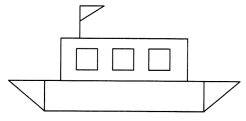(A) 3
(B) 4
(C) 5
(D) 6
Explanation:
The above picture has 3 squares.
The rest are 2 rectangles and 3 triangles.

Question 19.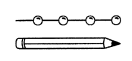The pencil is about _________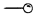long.
(A) 8
(B) 2
(C) 6
(D) 4
Option(D)
Explanation:
From the above information each onerepresent as 1 unit.
So, the pencil is about 4.

Short Answer Write the number in words.

Question 20.
11 ___________
Eleven
Explanation:
Expanded form or expanded notation is a way of writing numbers to see the math value of individual digits.
When numbers are separated into individual place values they can also form a mathematical expression.
10 + 1 = 11
It can also be written in words as,
Eleven

Question 21.
87 ____________
Eighty Seven
Explanation:
Expanded form or expanded notation is a way of writing numbers to see the math value of individual digits.
When numbers are separated into individual place values they can also form a mathematical expression.
80 + 7 = 87
It can also be written in words as,
Eighty Seven.

Write the number.

Question 22.
fifty-four ___________
Explanation:
Expanded form or number in words are separated into individual place values.
50 + 4 = 54

Question 23.
twenty-six ____________
Explanation:
Expanded form or number in words are separated into individual place values.
20 + 6 = 26

Look at the pictures. Then fill in the blanks.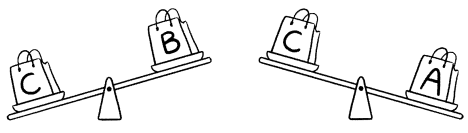Question 24.
Order the bags from lightest to heaviest.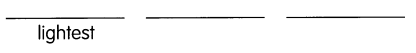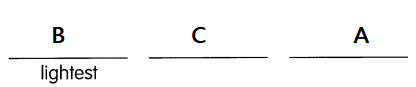Explanation:
When C and B weighed B is lighter than C.
When C and A weighed C is lighter than A.
When compared all the 3 weights the order of lightest is BCA.

Look at the picture. Then fill in the blanks. Use Ο as 1 unit.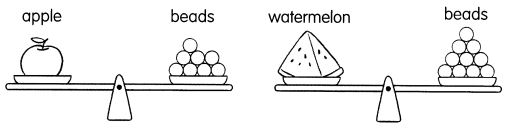Question 25.
The weight of the apple is about ___________ units.
Explanation:
From the above given information 1 unit is represented as O.
So, the weight of the apple is about O units.

Question 26.
The weight of the watermelon is about ___________ units.
Explanation:
From the above given information 1 unit is represented as O.
So, the weight of the watermelon is about O units.

Question 27.
The ___________ is heavier than the _________.
The beads are heavier than the watermelon.
Explanation:
From the above given information 1 unit is represented as O.
The weight of the watermelon and apple is about O units.
The weight of beads are 11 units.
The beads are heavier than the watermelon.

Look at each picture. Then write the time.

Question 28.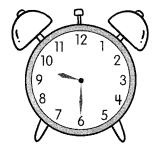9:30
Explanation:
In Clock there are 2 hands,
one is short hand denotes hours and long hand denotes minutes.
In the above clock the short hand is between 10 and 9
Long hand is on 6.
So the time is 9:30

Question 29.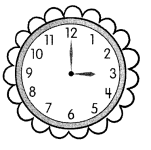3’O clock
Explanation:
In Clock there are 2 hands,
one is short hand denotes hours and long hand denotes minutes.
In the above clock the short hand is on 3 and long hand is on 12.
So the time is 3’O clock

Question 30.
Look at the picture. Then write the number in words.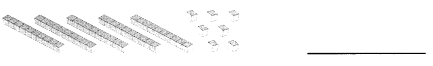Explanation:
The above picture has 5 bars which represents 10 blocks in each bar.
7 individual blocks.
50 + 7 = 57

Question 31.
Order the numbers from greatest to least.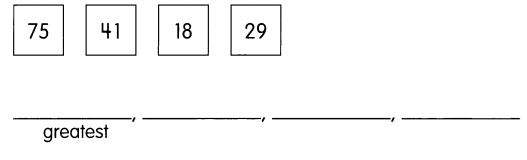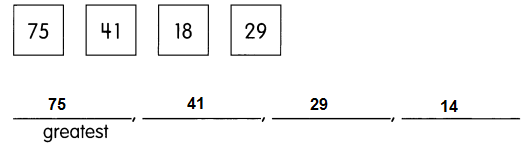Explanation:
Arrange the given numbers from greatest to least or ascending order.
Ascending order means big to small.
75, 41, 29, 14

Circle the two numbers that add up to 4 tens and 4 ones.

Question 32.
24 27 51 30 17
27 and 17
Explanation:
If we add 27 and 17 we get 4 tens and 4 ones.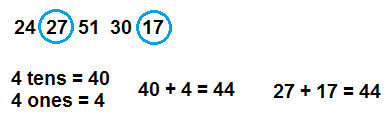Add the 2nd and 5th numbers.

Question 33.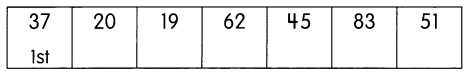Explanation:
In the given table the numbers are arranged in order,
like 1st number 37, 2nd number 20, 3rd number 19,
4th number 62, 5th number 45, 6th number 83 and 7th number 51.
By adding 2nd and 5th numbers,
we get 20 + 45 = 65

Question 34.
Subtract 6 tens 4 ones from 8 tens 2 ones.
Explanation:
6 tens = 60 and 4 ones = 4
60 + 4 = 64
8 tens = 80 and 2 ones = 2
80 + 2 = 82
Subtract 6 tens 4 ones from 8 tens 2 ones.
82 – 64 = 18

Name the shape that is shaded.

Question 35.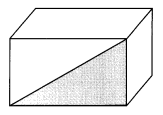Right Angle
Explanation:
When two straight lines intersect each other at 90˚ or perpendicular to each other at the intersection,
they form the right angle.
A right angle is represented by the symbol ∟.

Look at the number line. Fill in the missing numbers.

Question 36.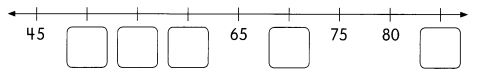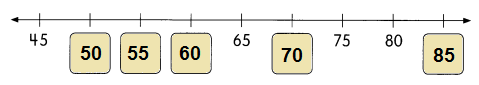Explanation:
n = 45
45 + 5 = 50
50 + 5 = 55
55 + 5 = 60
60 + 5 = 65
65 + 5 = 70
70 + 5 = 75
75 + 5 = 80
80 + 5 = 85

Look at the picture. Circle the correct word.

Question 37.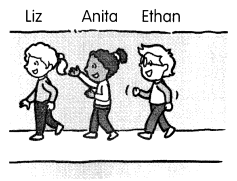a. Liz is first __________ last from the left.
Liz is first  from the left and Ethan is  last from the left.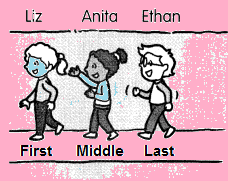b. Ethan is in front of __________ behind Anita.
Liz in front of Anita and Ethan behind Anita.
Explanation:
From the picture given above all the 3 friends are standing one after the other.
First is Liz, second is Anita and 3rd is Ethan.
So, Liz is first  from the left and Ethan is  last from the left.
Liz in front of Anita and Ethan behind Anita.

Extended Response

The picture graph shows the favorite food of 12 children.

Question 38.
Favorite Food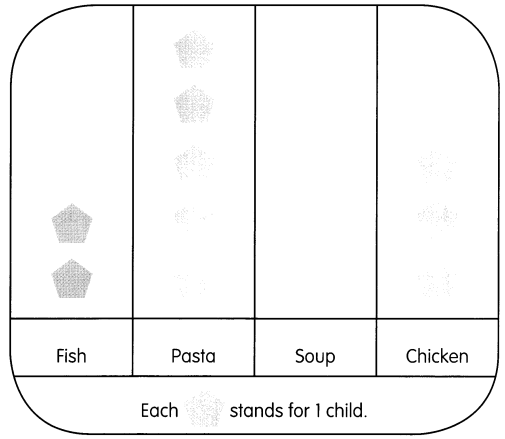Solve.
a. The same number of children named fish and soup as their favorite food.
Draw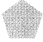to show the number of children who like soup.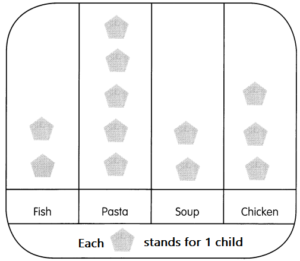Explanation:
Total number of children 12.
Number of children liked fish as their favorite food are 2.
From the given information the same number of children named fish and soup as their favorite food.
2 children likes soup.

b. ____________ is the favorite food for the most children.
Pasta is the favorite food for the most children.
Explanation:
Total number of children 12.
From the above given information,
2 children liked fish as their favorite food.
2 children liked Soup as their favorite food.
3 children liked chicken as their favorite food.
7 children liked other food as their favorite food.
Most of the children like Pasta as their favorite food.
so, 12 – 7 = 5

Ten families live on the same street. Every family has one pet. The picture shows their pets.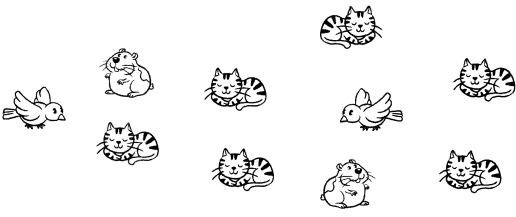Complete the tally chart to show the pet data. Then answer the questions.

Question 39.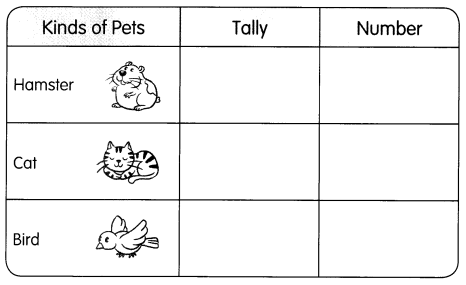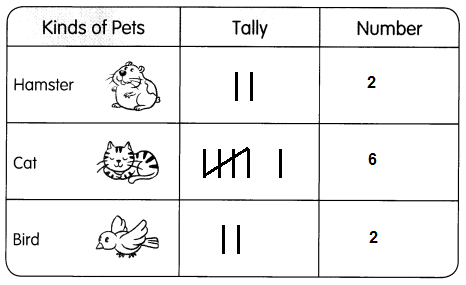a. How many pets are there in all?
b. Which pet has the greatest number?
6 cats are there

Make a bar graph using the data from the tally chart.

c.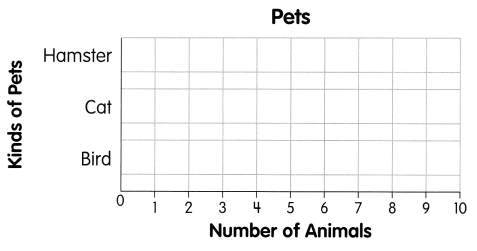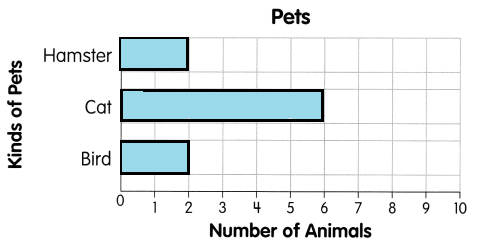Explanation:
From the above tally chart there are 2 Hamsters, 6 Cats and 2 Birds.
Total pets are 10.
Mot of the families have cats as pets.

Solve.

Question 40.
Sam has 32 marbles He puts all the marbles in bags. He puts 4 marbles in each bag. How many bags does he use?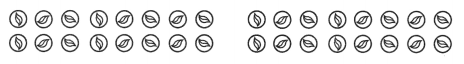He uses _____________ bags.